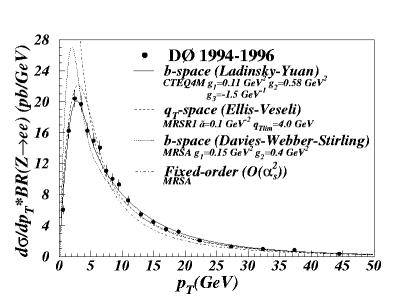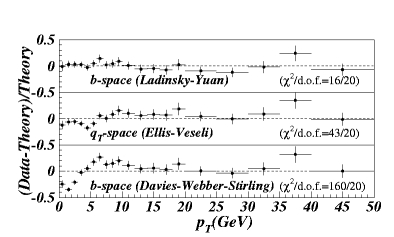# Transverse Momentum of the Z Boson## Publications and Documents:## "Plain English" discussion of the Z pT measurement

Making a Z boson
The Z boson is the neutral carrier of the weak force (the W+ and W- are the other two, charged, carriers). The DØ experiment is located at a proton-antiproton collider called the Tevatron. In proton-antiproton collisions, Z bosons are sometimes created when a quark from the proton collides with an antiquark from the antiproton. Transverse momentum is momentum (motion) perpendicular to the colliding beams. Because momentum is conserved, we know that if the quarks always collided head-on, the Z boson created would never have any transverse momentum. The fact that the Z boson can have lots of transverse momentum means that the quarks do not collide head-on, but bang into one another at some angle. Measuring the transverse momentum of the Z, therefore, tells us something about how quarks move around inside protons and antiprotons -- tells us something about the structure matter.

Seeing a Z boson
In an experiment we don't actually observe the Z boson itself because it decays too quickly -- it doesn't even travel as far as one atom! However, it does decay into electrons, muons, and quarks, which don't decay and are easy to observe in a detector like DØ. Most often, the Z decays to quarks, which are observed in the detector as a spray of particles called a jet. However, it is very difficult to observe Z boson decays into two jets if the Z is produced by colliding quarks (i.e., at a proton-antiproton collider) because the most commonly occuring process when colliding quarks is "dijet" production -- two quarks colliding with two quarks coming out. The probability of a Z boson being produced when two quarks collide is many 1000 times less than that of two quarks being produced. In the end, the rate for dijets is so high that one simply cannot tell the difference between two jets that came from a Z decay and two jets that came from two colliding quarks. I chose to study the "electron channel", where the Z boson decays into an electron and a positron, because electrons are the most precisely measured objects at DØ and would, therefore, yield the most precisely measured Z bosons.

More to come.